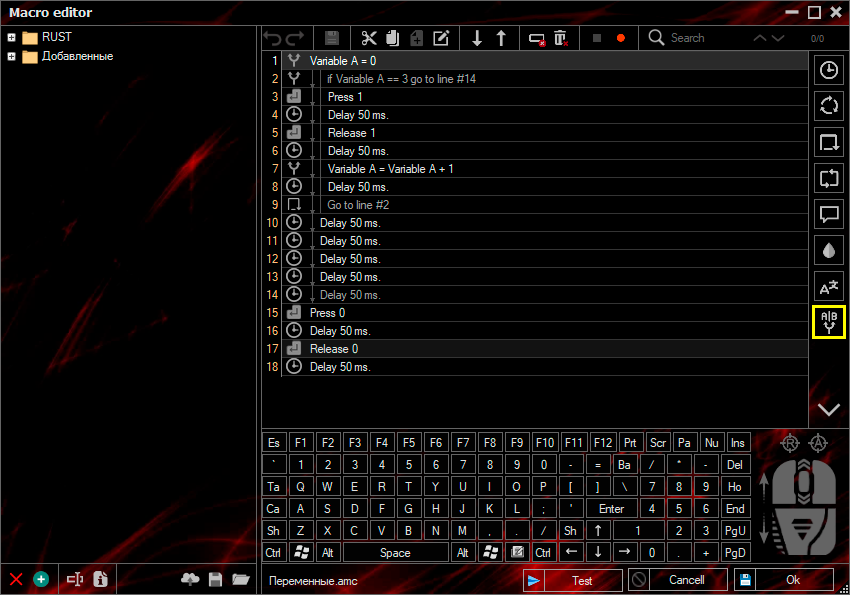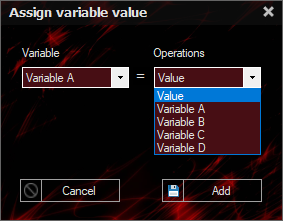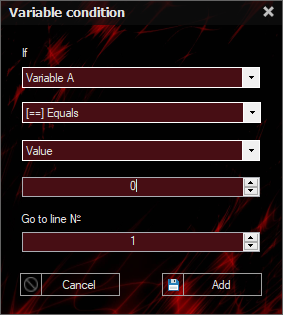Variables

## Creating a macro

### Variables

A variable function, is a mathematical object that occupies some set of numeric values. In a program, variables are used as mathematical expressions (add, subtract, divide, multiply). Simply put, variables act as "counters". There are two options in the function itself, namely setting a variable and checking it.There is nothing complicated about setting a variable, because there are a number of preset variables (a,b,c,d) with which all further operations are performed.The value "=" assigns a value to the variable on the left, which we enter ourselves, but if we expose a variable, the actions of the variable on the right will be transferred to the variable on the left, that is, the variable "a" = variable "a + 1" with initially 0 value "a" will give us the variable "a = 0 + 1 = 1", the same will be true for the other variable.

Conditions are created in the variable comparison. If [variable to be controlled] [condition: more/less/equal/not equal] [value/variable] then go to the line specified in the settings.by Termorey

### Video lesson

© 2016 - 2023 Keyran - program for creating and running macros | User agreement | Site Map